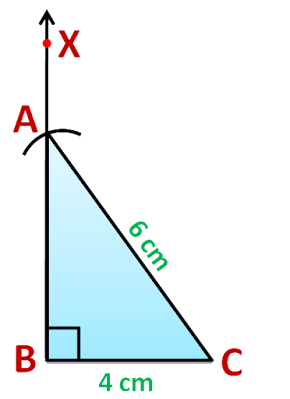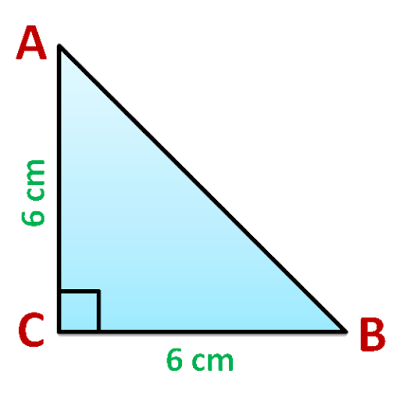# vs.eyeandcontacts.com

## Chapter 10 Practical Geometry Exercise 10.5

Question 1: Construct the right angled ΔPQR, where m∠Q = 90°, QR = 8cm and
PR = 10 cm.

Rough FigureActual FigureSteps of Construction
1. Draw QR = 8 cm.
2. Take Q as a centre draw an angle Q such that ∠XQR = 90°.
3. Take R as a centre, 10 cm as radius draw an arc to intersect QX at P.
4. Join PR.
5.   ⃤   PQR is our required triangle.

Question 2: Construct a right-angled triangle whose hypotenuse is 6 cm long and one of the legs is 4 cm long
Rough Figure

Actual FigureSteps of Construction
1. Draw BC = 4 cm.
2. Take B as a centre draw an angle B such that ∠XBC = 90°.
3. Take C as a centre, 6 cm as radius draw an arc to intersect BX at A.
4. Join AC.
5.   ⃤   ABC is our required triangle.

Question 3: Construct an isosceles right-angled triangle ABC, where m∠ACB = 90° and AC = 6 cm.
Rough FigureActual FigureSteps of Construction
1. Draw CB = 6 cm.
2. Take C as a centre draw an angle C such that ∠XCB = 90°.
3. Take C as a centre, 6 cm as radius draw an arc to intersect CX at A.
4. Join AB.
5.   ⃤   ABC is our required triangle.